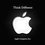# Fractions

There exists a interesting relationship between $27$ and $37$

$\frac{1}{27}=0.037037037...$ and $\frac{1}{37}=0.027027027...$ (That is, each number forms the other number's repeating decimal.)

This can be explained as follows :

Say $\frac{1}{X}=0.YYY....$ If $Y$ is n digits long,

then the fraction equals $Y$ times $10^{-n}+10^{-2n}+10^{-3n}...= \frac{Y}{(10^n-1)}$.

Therefore, we need $XY=10^n-1$. In the above case, $27*37=10^3-1$; other examples are $3*3=10^1-1$

So $\frac{1}{3}=0.333...$

or $11*9=10^2-1$ so $\frac{1}{11}=0.090909...$ and $\frac{1}{9}=0.11111...$ and so on.Note by Danish Ahmed
5 years, 5 months ago

This discussion board is a place to discuss our Daily Challenges and the math and science related to those challenges. Explanations are more than just a solution — they should explain the steps and thinking strategies that you used to obtain the solution. Comments should further the discussion of math and science.

When posting on Brilliant:

• Use the emojis to react to an explanation, whether you're congratulating a job well done , or just really confused .
• Ask specific questions about the challenge or the steps in somebody's explanation. Well-posed questions can add a lot to the discussion, but posting "I don't understand!" doesn't help anyone.
• Try to contribute something new to the discussion, whether it is an extension, generalization or other idea related to the challenge.

MarkdownAppears as
*italics* or _italics_ italics
**bold** or __bold__ bold
- bulleted- list
• bulleted
• list
1. numbered2. list
1. numbered
2. list
Note: you must add a full line of space before and after lists for them to show up correctly
paragraph 1paragraph 2

paragraph 1

paragraph 2

[example link](https://brilliant.org)example link
> This is a quote
This is a quote
    # I indented these lines
# 4 spaces, and now they show
# up as a code block.

print "hello world"
# I indented these lines
# 4 spaces, and now they show
# up as a code block.

print "hello world"
MathAppears as
Remember to wrap math in $$ ... $$ or $ ... $ to ensure proper formatting.
2 \times 3 $2 \times 3$
2^{34} $2^{34}$
a_{i-1} $a_{i-1}$
\frac{2}{3} $\frac{2}{3}$
\sqrt{2} $\sqrt{2}$
\sum_{i=1}^3 $\sum_{i=1}^3$
\sin \theta $\sin \theta$
\boxed{123} $\boxed{123}$

Sort by:

!!!

- 5 years, 5 months ago

It is Fantastic! Thanks for sharing @Danish Ahmed

- 5 years, 5 months ago

nice

- 5 years, 5 months ago

Excellent

- 5 years, 5 months ago

Oh that's nice! Thanks for sharing this pattern that you noticed.

Staff - 5 years, 5 months ago